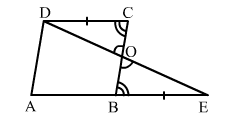# In the adjoining figure, ABCD is a parallelogram in which AB is produced to E so that BE = AB.

Question:

In the adjoining figure, ABCD is a parallelogram in which AB is produced to E so that BE = AB. Prove that ED bisects BC.Solution:

In ∆ODC and ∆​OEB, we have:
DC = BE                                   (∵ DC = AB)
∠COD = ∠BOE                        (Vertically opposite angles)
OCD = ∠OBE                        ( Alternate interior angles)

i.e., ∆​ODC ≅ ∆​OEB
⇒ OC = OB                                 (CPCT)
We know that BC = OC + OB.
∴ ED bisects BC.